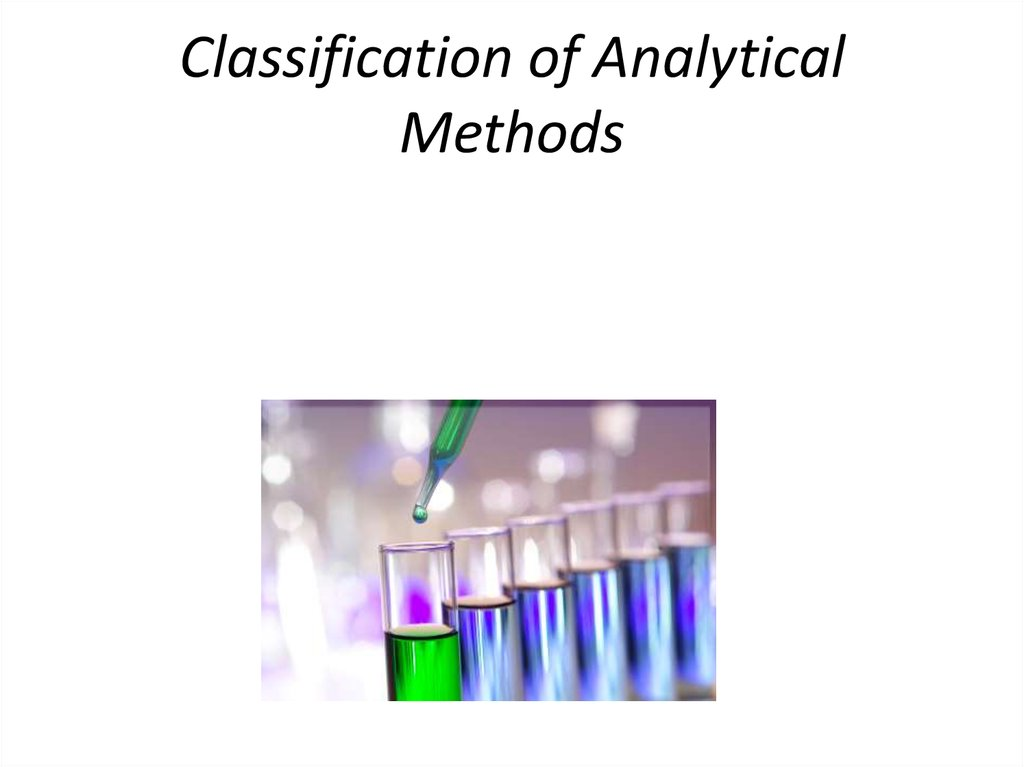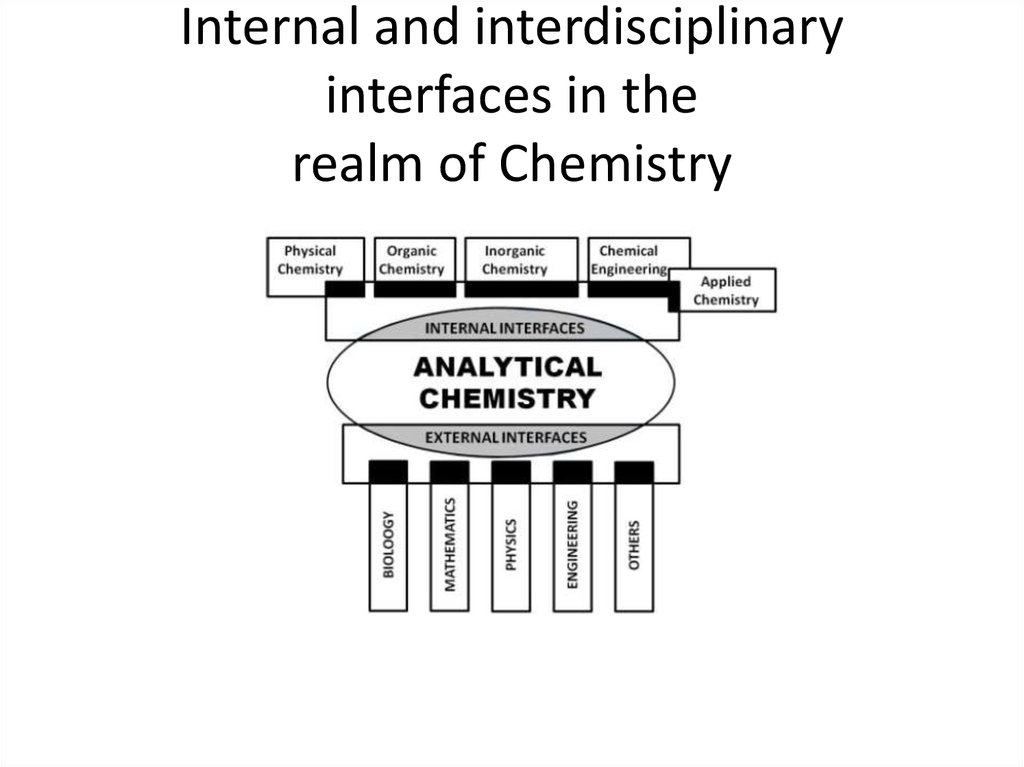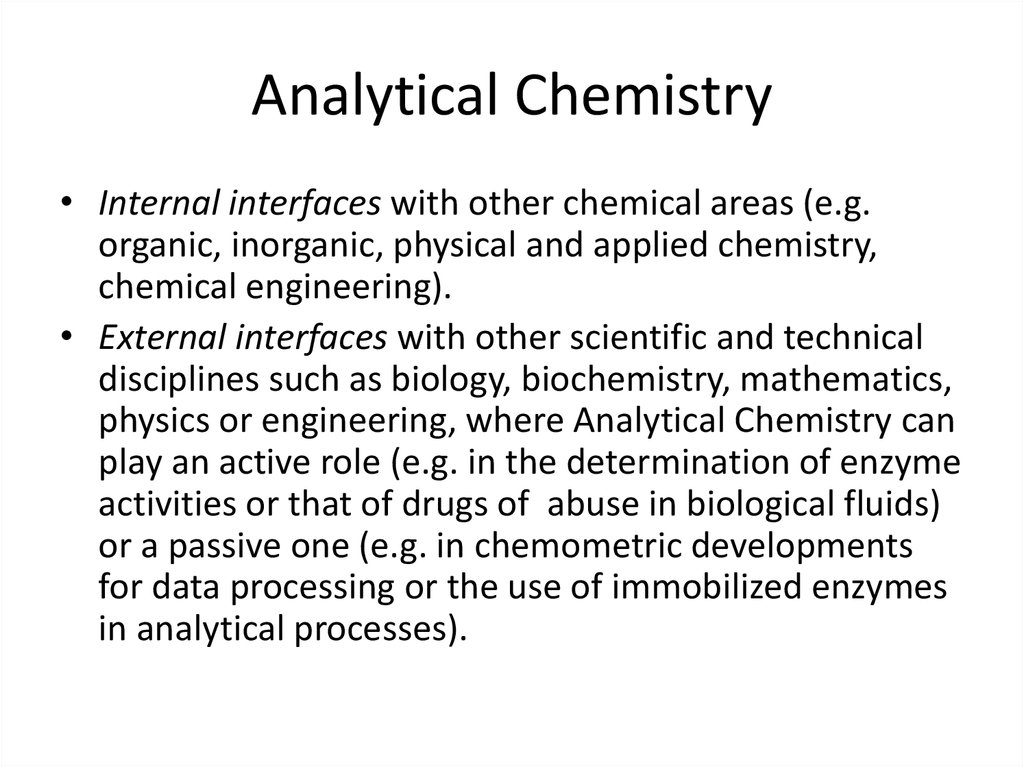# Classification of Analytical Methods

## 3. Analytical Chemistry

• Internal interfaces with other chemical areas (e.g.
organic, inorganic, physical and applied chemistry,
chemical engineering).
• External interfaces with other scientific and technical
disciplines such as biology, biochemistry, mathematics,
physics or engineering, where Analytical Chemistry can
play an active role (e.g. in the determination of enzyme
activities or that of drugs of abuse in biological fluids)
or a passive one (e.g. in chemometric developments
for data processing or the use of immobilized enzymes
in analytical processes).

## 4. Analytical Chemistry

• the discipline in charge of “Analysis” (the fourth
component of Chemistry in addition to Theory,
Synthesis and Applications, all of which are
mutually related via the vertices of the
tetrahedron in Figure
• the discipline in charge of the production of so
named “(bio)chemical information” or “analytical
information”; the discipline of (bio)chemical
measurements; and the chemical metrological
discipline, which is related to the previous
definition.

## 6. Definitions:

• “Analytical Chemistry is a scientific discipline that
develops and applies methods, instruments and
strategies to obtain information on the composition
and nature of matter in space and time” (Working
Party on Analytical Chemistry of the European
Federation of ChemicalSocieties).
• “Analytical Chemistry is a metrological discipline that
develops, optimizes and applies measurement
processes intended to produce quality (bio)chemical
information of global and partial type from natural and
artificial objects and systems in order to solve
analytical problems derived from information needs”.

## 7. Analytical Chemistry has two essential aims.

• the obtainment of as high metrological quality
as possible (i.e. of as true as possible nalytical
information with as low as possible
uncertainty).
• solving analytical problems derived from
(bio)chemical information needs posed by
“clients” engaged in a great variety of
activities (health, general and agrifood
industries, the environment).

## 8. Quality indicators

Top or capital analytical properties
• Accuracy
• Reliability
• Representativeness
Basic analytical properties
• Precision
• Sensitivity
• Selectivity
Productivity-related properties
• Expeditiousness
• Cost-effectiveness
• Personnel-related factors

## 9. Classification of techniques

• by the type of analytical technique – classical
or instrumental techniques;
• by the nature of the measurement data
generated – single-channel or multi-channel
techniques;
• by the quantitation method (by which the
analyte concentration is calculated) – relative
or absolute techniques.

## 10. Basic Equipment and Instrumentation

beaker
Photo of a typical electronic balance.

Proper means of
meniscus on a
or pipet.
dropping pipet

## 12. Classical Methods:

Separation of analytes by precipitation, extraction, or distillation.
Qualitative analysis by reaction of analytes with reagents that yielded products
that could be recognized by their colors, boiling or melting points, solubilities,
optical activities, or refractive indexes.
• Quantitative analysis by gravimetric or by titrimetric techniques.
In the early years of chemistry, most analyses were carried out by separating
components of interest in a sample by precipitation, extraction, or distillation. For
quantitative analyses, the separated components were then treated with reagents
that yielded products that could be recognized by their colors, boiling points or
melting points, their solubility in a series of solvents, their odors, their optical
activities, or their refractive indexes. For quantitative analyses, the amount of
analyte was determined by gravimetric or by titrimetric measurement.
• Gravimetric Methods – the mass of the analyte or some compound produced from
the analyte was determined.
• Titrimetric Methods – the volume or mass of a standard reagent required to react
completely with the analyte was measured.

## 13. Titrimetric Analysis

The term titrimetric analysis refers to
quantitative chemical analysis carried out by
determining the volume of a solution of
accurately known concentration which is
required to react quantitatively with a measured
volume of a solution of a substance to be
determined. The solution of accurately known
concentration is called standard solution

## 14. For use in titrimetric analysis a reaction must have the following conditions

1- There must be a simple reaction which can be expressed by
a chemical equation; the substance to be determined should
react completely with the reagent instoichiometric or
equivalent properties.
2- The reaction should be relatively fast. (Most ionic reaction
satisfy this condition.) In some cases the addition of a catalyst
may be necessary to increase the speed of areaction.
3- There must be an alteration in some physical or chemical
property of the solution at the equivalence point.
4- An indicator should be available which, by a change in
physical properties (color or formation of a precipitate),
should sharply define the end point of the reaction.

## 15. Definition of some terms

• Titration is the process in which the standard reagent
is added to a solution of ananalyte until the reaction
between the analyte and reagent is complete.
• Equivalence point and End point - The equivalence
point of a titration is a theoretical point that can not be
determine experimentally. Instead, we can only
estimate its position by observing some physical
change associated with the condition of equivalence.
This change is called the end point for titration.
• Titration error - The difference between the observed
end point and the true equivalence point in a titration.

## 16. Indicators

• Indicators are often added to analyte solution in order to give
an observable physical change (end point) at or near the
equivalence point. In other wards indicator is a compound
having a physical property (usually color) that changes
abruptly near thee quivalence point of a chemical reaction.

## 18. Classification of reaction in titrimetric analysis

1.
Neutralization reaction, or acidimetry and alkalimetry. These include thetitration of free bases,
or those formed from salts of weak acids by hydrolysis with astandard acid (acidimetry), and the
titration of free acids, or those formed by thehydrolysis of salts or weak bases, with a standard
base (alkalimrtry). The reactioninvolve the combination of hydrogen and hydroxide ions to form
water. Also under this heading must be included titrations in non-aqueous solvents, most of
whichinvolve organic compounds.
2. Precipitation reaction.
These depend upon the combination of ions to form asimple precipitate as in the titration of silver ion
with solution of chloride. No changein oxidation state occurs.
3. Complex formation reaction.
These depend upon the combination of ions, other than hydrogen or hydroxide ion, to form a soluble
slightly dissociated ion or compound, as in the titration of a solution af a cyanide with silver
nitrate.Ethylendiaminetera-acetic acid, largely as the disodium salt of EDTA, is a veryimportant
reagent for complex formation titration and has become on of the mostimportant reagents used
in titrimetric analysis.
4. Oxidation-reduction reaction.
Under this heading are included all reactionsinvolving change in oxidation number or transfer of
electrons among the reactivesubstance. The standard solutions are either oxidizing or reducing
agents.

## 19. Instrumental Methods:

Measurements of physical properties of analytes, such as
conductivity,
electrode potential,
light absorption,
or emission, mass to charge ratio,
and fluorescence,
began to be used for quantitative analysis of a variety of
inorganic, organic, and biochemical analyte. Highly efficient
chromatographic and electrophoretic techniques began to
replace distillation, extraction, and precipitation for the
separation of components of complex mixtures prior to their
qualitative or quantitative determination. These newer
methods for separating and determining chemical species are
known collectively as instrumental methods of analysis.

## 20. The advantages of instrumental methods over classical methods include:

1. The ability to perform trace analysis, as we have
mentioned.
2. Generally, large numbers of samples may be
analyzed very quickly.
3. Many instrumental methods can be automated.
4. Most instrumental methods are multi-channel
techniques (we will discuss these shortly).
5. Less skill and training is usually required to
perform instrumental analysis than
classicalanalysis.

## 21. Instrumental analysis can be further classified according to the principles by which the measurement signal is generated

• Electrochemical methods of analysis
• Spectrochemical methods of analysis
• mass spectroscopy
• chromatography and electrophoresis.
• electrogravimetry, and potentiostatic
amperostatic coulometry

## 22. Lists the names of instrumental methods that are based upon various analytical signals.

Signal
Instrumental Methods
Emission spectroscopy (X-ray, UV, visible, electron, Auger); fluorescence,
phosphorescence, and luminescence (X-ray, UV, and visible)
Spectrophotometry and photometry (X-ray, UV, visible, IR); photoacoustic
spectroscopy; nuclear magnetic resonance and electron spin resonance
spectroscopy
Turbidimetry; nephelometry; Raman spectroscopy
Refractometry; interferometry
X-Ray and electron diffraction methods
Polarimetry; optical rotary dispersion; circular dichroism
Electrical potential
Potentiometry; chronopotentiometry
Electrical charge
Coulometry
Electrical current
Polarography; amperometry
Electrical resistance
Conductometry
Mass-to-charge ratio
Mass spectrometry
Rate of reaction
Kinetic methods
Thermal properties
Thermal conductivity and enthalpy
Activation and isotope dilution methods

## 23. Electrochemical methods of analysis

• in which the analyte participates in a redox reaction or
other process.
In potentiometric analysis, the analyte is part of a galvanic
cell, which generates a voltage due to a drive to
thermodynamic equilibrium. The magnitude of the voltage
generated by the galvanic cell depends on the concentration
of analyte in the sample solution.
In voltammetric analysis, the analyte is part of an electrolytic
cell. Current flows when voltage is applied to the cell due to
the participation of the analyte in a redox reaction; the
conditions of the electrolytic cell are such that the magnitude
of the current is directly proportional to the concentration of
analyte in the sample solution.

## 24. Spectrochemical methods of analysis

• in which the analyte interacts with electromagnetic
radiation. Most of the methods in this category are
based on the measurement of the amount of light
absorbed by a sample; such absorption-based
techniques include atomic absorption, molecular
absorption, and nmr methods.
• The rest of the methods are generally based on the
measurement of light emitted or scattered by a
sample; these emission-based techniques include
atomic emission, molecular fluorescence, and Raman
scatter methods.

## 25. The technique of mass spectroscopy

• in which the analyte is ionized and subsequently
detected. Although in common usage, the term
“spectroscopy” is not really appropriate to
describe this method, since electromagnetic
radiation is not usually involved in mass
spectroscopy. Perhaps the most important use of
mass spectrometers in quantitative analysis is as
a gas or liquid chromatographic detector. A more
recent innovation is the use of an inductively
coupled plasma (ICP) as an ion source for a mass
spectrometer; this combination (ICP-MS) is a
powerful tool for elemental analysis.

## 26. Single-Channel vs Multi-Channel Techniques

• single-channel techniques will generate but a single number for
each analysis of the sample. Examples include gravimetric and
potentiometric analysis. In the former, the signal is a singlemass
measurement (e.g., mass of the precipitate) and in the latter
method the signal is a single voltage value.
• • multi-channel techniques will generate a series of numbers for a
single analysis. Multi-channel techniques are characterized by the
ability to obtain measurements while changing some independently
controllable parameter. For example, in a molecular absorption
method, an absorption spectrum may be generated, in which the
absorbance of a sample is monitored as a function of the avelength
of the light transmitted through the sample. Measurement of the
sample thus produces a series of absorbance values.

## 27. Multi-channel methods have two important advantages over their single-channel counterparts:

1. They provide the ability to perform
multicomponent analysis. In other words, the
concentrations of more than one analyte in a single
sample may be determined.
2. Multi-channel methods can detect, and
sometimes correct for, the presence of a number of
types of interferences in the sample. If uncorrected,
the presence of the interference will result in biased
estimates of analyte concentration.

## 28. Relative vs Absolute Techniques

• Another way of classifying analytical techniques is
according to the method by which the analyte
concentration is calculated from the data:
• in absolute analytical techniques, the analyte
concentration can be calculated directly from
measurement of the sample. No additional
measurements are required (other than
ameasurement of sample mass or volume).
• in relative analytical techniques, the measurement of
the sample must be compared to measurements of
additional samples that are prepared with the use of
analyte standards (e.g.,solutions of known analyte

## 29. The difference between absolute and relative techniques is that the latter requires additional measurements in order to obtain

an estimate of the analyte
concentration.

## 31. Calibration Curve Method

For any instrumental method used for quantitative
chemical analysis, there is some functional relationship
between the instrument signal, r, and the analyte
concentration, CA:
r = f(CA)
The calibration curve approach to quantitation is an
attempt to estimate the nature of this functional
relationship. A series of calibration standards are
analyzed, and a “best-fit” line or curve is used to describe
the relationship between the analyte concentration in the
calibration standards and the measured signal. The
following figure demonstrates the concept.

## 32. Typical calibration curve

The instrument response is
measured for a series of
calibration standards, which
contain a known concentration
of analyte. The curve is a
function that
describes the functional
relationship between signal and
concentration. Note that the
calibration
curve should never be
extrapolated (i.e., never
extended beyond the range of
the calibration
measurements).

• The central philosophy of the calibration curve method is this: the function
that describes the relationship between signal and concentration for the
calibration standards also applies to any other sample that is analyzed.
Any factor that changes this functional relationship will result in a biased
estimate of analyte concentration.
• A linear relationship between signal and concentration is desirable,
generally resulting in the best accuracy and precision using the fewest
number of calibration standards.
• Ideally, the analyte concentration should only be calculated by
interpolation, not by extrapolation. In other words, the analyte
concentration should be within the range of concentrations spanned by
the calibration standards. If the analyte concentration in thesample is too
great, then the sample may be diluted. If the analyte concentration is too
small, then additional calibration standards can be prepared. For best
precision, the concentration is close to the mean concentration of the
calibration standards.

## 34. Electroanalytical methods

• Electroanalytical methods are a class of techniques in
analytical chemistry which study an analyte by
measuring the potential (volts) and/or current
(amperes) in an electrochemical cell containing the
analyte. These methods can be broken down into
several categories depending on which aspects of the
cell are controlled and which are measured. The three
main categories are potentiometry (the difference in
electrode potentials is measured), coulometry (the
cell's current is measured over time), and voltammetry
(the cell's current is measured while actively altering
the cell's potential).

## 35. Potentiometry

• Potentiometry passively measures the potential of a
solution between two electrodes, affecting the solution
very little in the process. The potential is then related
to the concentration of one or more analytes. The cell
structure used is often referred to as an electrode even
though it actually contains two electrodes: an indicator
electrode and a reference electrode (distinct from the
reference electrode used in the three electrode
system). Potentiometry usually uses electrodes made
selectively sensitive to the ion of interest, such as a
fluoride-selective electrode. The most common
potentiometric electrode is the glass-membrane
electrode used in a pH meter.